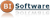imieniny: Błażej, Margerita

Osób online: 1

## Krawczak, Multilayer

In this book several multilayer neural networks are treated as multistage systems, and learning process is considered as a particular sort of multistage optimal control problem.

On the base of dynamic programming methodology, and by introducing the gain parameter to the neuron models, several learning algorithms were de­veloped, e.g. the first order differential dynamic programming algorithm and the heuristic dynamic programming algorithm.

Additionally, the updating of learning meant as multiobjective optimisa­tion problems is developed, the approach allows for decreasing learning time.

The development of the adjoint neural networks yields direct expres­sions of gradient based learning algorithms.

In the second part of the book the Generalized Net theory was used for modelling of neural networks processes. First, the Generalized Net models of the simulation processes, and next the Generalized Net models of learning algo­rithms developed in this book were introduced and discussed. Also, the Gener­alized Net model of the adjoint neural networks was discussed.

The author of this book works for the Systems Research Institute of the Polish Academy of Sciences as well as for the Warsaw School of Information Technology (as the Vice-rector).

Strona główna
﻿